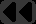## Oskar Schirmer### 2006

1002PostScript font encoding in unicode times

### 2009

0502evolution in the context of omnipotence

### 2010

1209Zur Frage des Rechtes auf Freigabe geheimgehaltener Informationen

### 2011

0308similar words search algorithm
The task of the following algorithm is to search for similar words in a given corpus. Two words are similar if it is possible to transform the one word into the other through a given maximum number n of simple transformations. A simple transformation is one of the following:
• One character deleted
• One character replaced by an other

First, prepare the corpus to have each word in it be referenced through a bunch of hash values by generating a list of sketches for each word, then calculate the hash value for each sketch and let it refer to the proper word. A sketch is the word with zero or more, but at most n, characters in it replaced by a wildcard, e.g. “.”. E.g. for the word fact and n=2 the list of sketches has eleven entries: fact, fac., fa.t, fa.., f.ct, f..t, f.c., .act, ..ct, .a.t, .ac.

Second, prepare the word to be searched. Generate a list of alterations by applying all simple transformations to all character positions within the word, with two restriction:

• Do not add arbitrary characters, but only wildcards “.”.
• Do apply at most n simple transformations.
E.g. for the word cat and n=2 the list of alterations is: ..at, ..cat, ..t, .a., .a.t, .a, .act, .at., .at, .c.at, .c.t, .ca., .ca.t, .ca, .cat., .cat, .ct, .cta, .t, .ta, a., a.t, a, ac., ac.t, ac, act., act, at., at, c.., c..at, c..t, c., c.a., c.a.t, c.a, c.at., c.at, c.t., c.t, c.ta, c, ca.., ca..t, ca., ca.t., ca.t, ca, cat.., cat., cat, ct., ct, cta., cta, t, ta

Now, for each alteration, calculate the hash value. Use these hash values to find a sparse list of candidates to match against in the prepared corpus. In the given example, the seventh alteration, .act, will match the eighth sketch of fact.

Note 1: Theoretically, the first two simple transformations are sufficient to define some similarity, but in reality the latter two represent common typos in written text, so they are useful when it is about finding typos.

Note 2: The algorithm requires similarity to be restricted to a fixed number of simple transformations before preparing the corpus. Thus, it is not suited for search at ad hoc defined similarity.

Note 3: With n=2, the number of sketches for a word with k characters is k(k+1)/2 + 1.

Note 4: With n=2, and 4 simple transformations, an upper limit for the number of alterations for a word with k characters is 8k(k-1) + 10. Omitting the fourth simple transformation (jumbled letters) this reduces to (9k+1)k/2 + 4.

0411a thought on dictionary type implementation with PostScript

### 2012

0405hardware event handling using the XMOS example

### 2023

0808interrupts dispensable on million core system